Sorry, you need to enable JavaScript to visit this website.

# Bayesian network: An Introduction in Software Testing

View Tags

## Bayesian network: An Introduction in Software Testing November 30, 2015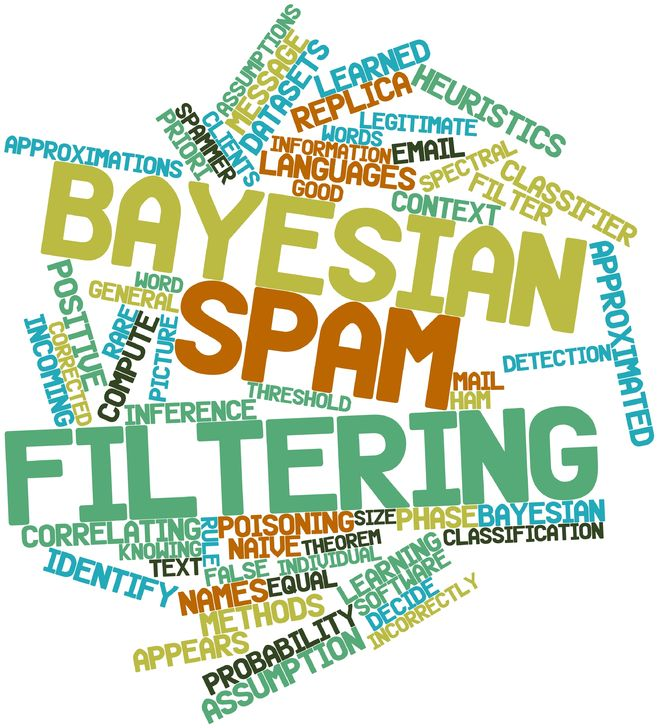Bayesian networks (BNs) are a progressively popular technology for software testing, cognitive Engineering and support systems, because probability plays a major role. A Bayesian network is a directed, acyclic graph whose nodes represent random variables and arcs represent direct dependencies. The arcs often, but not always, also represent direct causal connections between the variables. The nodes pointing to X are called its parents and collectively are denoted. The relationship between variables is enumerated by conditional probability tables (CPTs) associated with each node, namely). The CPTs collected efficiently represent the full joint distribution. The main objective of the method is to model the posterior conditional probability distribution of outcome (often causal) variable(s) after observing new evidence. Bayesian networks may be constructed either manually with knowledge of the underlying domain, or automatically from a large dataset by an appropriate software.

I will demonstrate with the design of a Bayesian Network for a simplified version of the following example in context of software testing. Suppose, we attempt to calculate DRE (Defect Removal Efficiency). We would like to know of the possible number of causes. Here we assume only two possible causes of this misfortune: Test efficiency and Test coverage.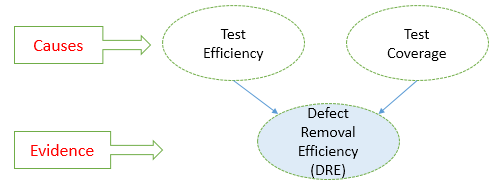Fig. 1: Directed acyclic graph representing two independent possible causes of a Defect removable efficiency

The first step is to decide what the variables of interest are, which will become the nodes in the BN. The two causes in this banal example are assumed to be independent (there is no edge between the two causal nodes), but this assumption is not necessary in general. Unless there is a cycle in the graph, Bayesian networks are able to capture as many causal relations as it is necessary to credibly describe the real-life situation.

The goal is to calculate the posterior conditional probability distribution of each of the possible unobserved causes given the observed evidence, i.e. P [Cause | Evidence]. However, in practice, we are often able to obtain only the converse conditional probability distribution of observing evidence given the cause, P [Evidence | Cause]. The whole concept of Bayesian networks is built on Bayes theorem, which helps us to express the conditional probability distribution of cause given the observed evidence using the converse conditional probability of observing evidence given the cause

P [Cause | Evidence] =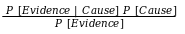1. Getting back to our example, we suppose that Test efficiency, denoted by TE and efficiency is “High” with probability 0.80, P [TE = High] = 0.80, and Test coverage, denoted by TC, coverage is “High” with probability 0.75, P [TC = High] = 0.75. It is reasonable to assume Test efficiency and Test coverage as independent. In other words, if DRE denotes the Defect Removable Efficiency, then the joint probability function and corresponding distribution value is

P [DRE, TC, TE] = P [DRE | TE, TC] P [TE] P [TC].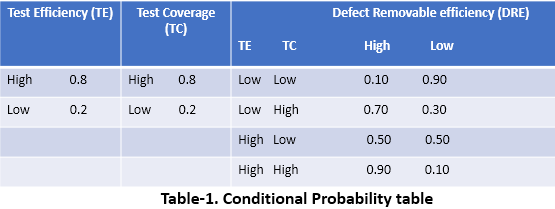2. In this setting, the probability of DRE consider Prior:

P (DRE = High) = 1 − P (DRE = Low) = 0.90

3. P [DRE = High] can be calculated as when TE is High and TC is High

P [DRE = High, TE = High, TC = High] = ∑ (P [DRE = High| TE, TC] · P [TE] · P [TC])= 0.90 x 0.80 x 0.75 = 0.54.

4. Posterior probability after observing Test efficiency and Test coverage is High: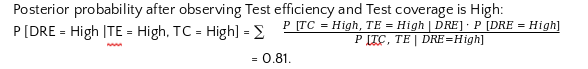5. The probability that the DRE is High, declines preliminary supposing that the prior of DRE is High (not as much as expected because of strong prior and unpredictable DRE). The model can response queries like “If TE is high, given the DRE is high then what the probability is?" Using the Bayes formula, we find P [TE = High| DRE = High] = ∑ P [TE = High, TC | DRE = High] = (0.54 + 0.10) / (0.54+0.10+0.0025+0.105) = 0.86.

6. Posterior after observing DRE is High, what the probability of TC is High: P [TC = High| DRE = High] = ∑ P [TE, TC = High| DRE = High]= 0.54 + 0.075) / (0.54+0.10+0.0025+0.105) = 0.82.

7. The probability that the Test coverage is High, increases from observing that the DRE is High.

Without making any observations, this BN tells us that the most likely distribution of Test efficiency is 86.0%, though the Test Coverage is most likely 82.0%.

In our second step, suppose that the Test coverage (TC) has a direct effect on the usage of the Test Efficiency (TE). Then the condition can be demonstrated with a Bayesian network (shown).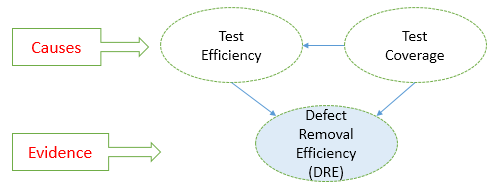Fig. 2: Directed acyclic graph. Test coverage influences Test efficiency and both Test coverage and Test efficiency influences Defect removable efficiency

All three variables have two possible values, High and Low.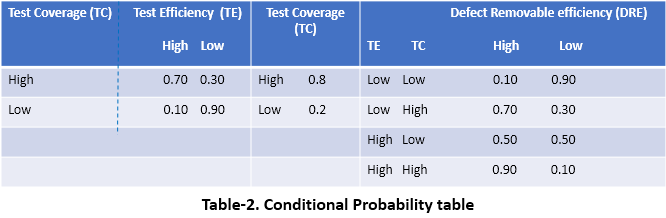Figure 2 and Table 2 shows a sample Bayesian network and conditional probability tables respectively when TE depends upon TC. The joint probability function and corresponding distribution value is P [DRE, TC, TE] = P [DRE| TE, TC] P [TE|TC] P [TC].

8. In this setting, the probability of DRE consider Prior: P (DRE = High) = 1 − P (DRE = Low) = 0.90.
9. P [DRE = High] can be calculated as when TE is High and TC is High P [DRE = High, TE = High, TC = High] = ∑ (P [DRE = High| TE, TC] · P [TE | TC] · P [TC])= 0.90 x 0.70 x 0.75 x 0.9 = 0.4725.
10. Posterior probability after observing Test efficiency and Test coverage is High:

P [DRE = High |TE = High, TC = High] =0.81.
11. Posterior after observing DRE is High, What the probability of TE is High:

P [TE = High| DRE = High] = 0.729323
12. Posterior after observing DRE is High, What the probability of TC is High:

P [TC = High| DRE = High] = 0.947368
13. As it can be seen in these examples of causal and indicative inferences, it is possible to propagate the effect of states of variables (nodes) to calculate posterior probabilities. Propagating the effects of variables to the successors, or analysing the probability of some predecessor variable based on the probability of its successor is very important in defect removable efficiency since software metrics are related to each other, and that is why the weight of a metric might be dependent on another metric based on this relationship.

• Uffe B. Kjærulff and Anders L. Madsen (2008): Bayesian Networks and Influence Diagrams: A Guide to Construction and Analysis
• Kevin B. Korb and Ann E. Nicholson (2010): Bayesian Artificial Intelligence